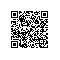# Python 命令行之旅：初探 argparse

## 前言

本系列文章默认使用 Python 3 作为解释器进行讲解。

## 介绍

argparse 作为 Python 内置的标准库，提供了较为简单的方式来编写命令行接口。当你在程序中定义需要哪些参数，argparse 便会从 sys.argv 中获取命令行输入进行解析，对正确或非法输入做出响应，也可以自动生成帮助信息和使用说明。

## 快速开始

### 设置解析器

import argparse
parser = argparse.ArgumentParser(
description='My Cmd Line Program',
)

### 定义参数

# 添加 nums 参数，在使用信息中显示为 num
# 其类型为 int，且支持输入多个，且至少需要提供一个
help='a num for the accumulator')
# 添加 --sum 参数，该参数被 parser 解析后所对应的属性名为 accumulate
# 若不提供 --sum，默认值为 max 函数，否则为 sum 函数
const=sum, default=max,
help='sum the nums (default: find the max)')

### 解析命令行

args = parser.parse_args(['--sum', '-1', '0', '1'])
print(args) # 结果：Namespace(accumulate=<built-in function sum>, nums=[-1, 0, 1])

### 业务逻辑

result = args.accumulate(args.nums)
print(result)  # 基于上文的 ['--sum', '-1', '0', '1'] 参数，accumulate 为 sum 函数，其结果为 0

### 代码梳理

# cmd.py
import argparse

# 1. 设置解析器
parser = argparse.ArgumentParser(
description='My Cmd Line Program',
)

# 2. 定义参数
help='a num for the accumulator')
const=sum, default=max,
help='sum the nums (default: find the max)')

# 3. 解析命令行
args = parser.parse_args()

# 4. 业务逻辑
result = args.accumulate(args.nums)
print(result)

$python3 cmd.py --sum -1 0 1 0 若我们需要对一组数字求最大值，只需执行： $ python3 cmd.py -1 0 1
1

\$ python3 cmd.py a b c
usage: cmd.py [-h] [--sum] num [num ...]
cmd.py: error: argument num: invalid int value: 'a'

usage: cmd.py [-h] [--sum] num [num ...]

My Cmd Line Program

positional arguments:
num         a num for the accumulator

optional arguments:
-h, --help  show this help message and exit
--sum       sum the nums (default: find the max)

## 小结使用钉钉扫一扫加入圈子
+ 订阅